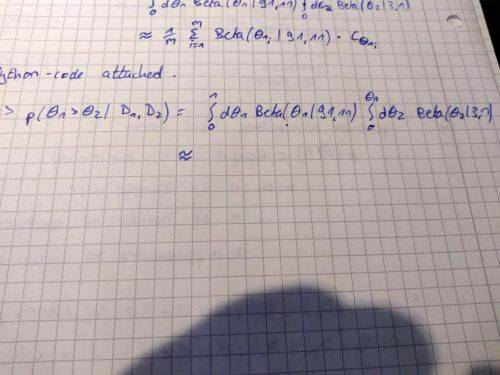# Numerical Integration

Greetings,

I am desparately trying to solve a double integral via Monte Carlo integration. I integrate over two probability densities, the Beta distributions. Python can easily draw samples from these densities and calculate its function values. The integral can be seen here:Now my idea was to sample n values ## \theta_1 ## from the first Beta function and insert it into the function.
For each ## \theta_1 ## I would then calculate the inner integral through sampling n ## \theta_2 ## from the second Beta function.
I would then calculate the entire integral via:
## \frac{1}{n} \sum_{i=1}^n ## Beta(##\theta_1^{i}## | 91,11) *## C_i##
with ##C_i=\frac{\theta_1^{i}}{m} \sum_{j=1}^m ## Beta(##\theta_2^{j}## | 3,1)

However, I could only consider those ## \theta_2 ## with ##\theta_2 < \theta_1 ##.
At first, I used an if-structure (commented part of my code) to store only the fitting m ##\theta_2## in a list for the calculation of the integral. But I figured that ##\theta_2 < \theta_1## is often not fulfilled, so the approximation of the inner integral would need much larger sample sizes. It did not lead to correct results.

Then I tried to approximate the inner integral through random sampling. For each ##\theta_1##, just sample n random ##\theta_2 \in (0, \theta_1) and approximate the integral with the described sum above.
But I get a result of 6.71...??
As a probability, the right result should be < 1. In fact, I know it to be around 0.7.

Can someone see my error?

Regards!

Python:
from scipy.stats import beta
import random

n=3000 #sample sizes

sample_theta1=[beta.rvs(91,11) for x in range(n)]

beta_values_theta1=beta.pdf(sample_theta1,91,11)

summands_total_integral=[]
sample_theta2=[]
beta_values_theta2=[]
integrals_theta2=[]

#calculate integral over theta2 for each theta1
for x in sample_theta1:
#    sample_theta2=[beta.rvs(3,1) for z in range(n)]
#    for z in range(n):
#        if sample_theta2[z]<x:
#            beta_values_theta2.append(beta.pdf(sample_theta2[z],3,1))
#    integrals_theta2.append(float(x) / len(beta_values_theta2) * sum(beta_values_theta2))
#    beta_values_theta2.clear()
sample_theta2=[random.uniform(0, x) for z in range(n)]
beta_values_theta2=beta.pdf(sample_theta2, 3, 1)
integrals_theta2.append(float(x) / n * sum(beta_values_theta2))

#calculate summands for approximation of theta1 integral
for x in range(n):
summands_total_integral.append(beta_values_theta1[x] * integrals_theta2[x])

result=float(1) / n * sum(summands_total_integral)
print(result)

## Answers and Replies

jedishrfu
Mentor
I would try printing out intermediate results and then stepping through to validate manually that they are correct.

This is the only way to see where things are going awry.

One other trick is to find a simpler known solution and step it through the algorithm to see where it goes off course.

The last trick would be to use parentheses around things the way you want them to be evaluated as sometimes our notion of how a computer does may be wrong ie different languages have differing conventions for more obscure operations.

As an example, the expression float(1) / n * sum(summands_total_integral):

Did you mean:

results=float(1) / (n * sum(summands_total_integral))

or

results=(float(1) / n) * sum(summands_total_integral)

The same question here:

integrals_theta2.append(float(x) / n * sum(beta_values_theta2))

Well, this would take ages. I thought there might be someone that sees immediately where the error is.

I tried it with random sampling Monte Carlo integration as well:
Just sample ##\theta_{1,2} \in (0,1) ## randomly for n times. Now anytime ##\theta_1 ## is greater than the respective ##\theta_2## in the other list, insert them into the respective function and multiply the values. Then sum over all of these products and divide by the number of fitting ##\theta##-pairs.
Still strange results. I feel like there is something wrong with my application of the method.
Python:
from scipy.stats import beta
import random

n=100000

#create samples (theta1, theta2) with theta1 > theta2
sample1=[beta.rvs(91,11) for x in range(n)]
sample2=[beta.rvs(3,1) for x in range(n)]
sample_2d_beta=[]

for x in range(n):
if sample1[x]>sample2[x]:
sample_2d_beta.append(beta.pdf(sample1[x],91,11) * beta.pdf(sample2[x],3,1))

result=(0.5 / len(sample_2d_beta)) * sum(sample_2d_beta)
print(result)

edit: I just realize I didn't do random sampling there, it's practically the same method as before, just without separating the integral in inner and outer integral.
With random sampling, it works just fine:
Python:
import random

n=1000000

#create samples (theta1, theta2) with theta1 > theta2
sample1=[random.uniform(0,1) for x in range(n)]
sample2=[random.uniform(0,1) for x in range(n)]
sample_2d_beta=[]

for x in range(n):
if sample1[x]>sample2[x]:
sample_2d_beta.append(beta.pdf(sample1[x],91,11) * beta.pdf(sample2[x],3,1))

result=(0.5 / len(sample_2d_beta)) * sum(sample_2d_beta)
print(result)
It gives the correct result of 0.71.

Still, I would like to understand where I am wrong in the importance sampling part from above. If anyone has a hint, I would appreciate it.

Last edited:
Ahh, I got my own error.

Importance sampling does not work that way. If I take my sample directly from the distribution that forms the integrand, I must not insert them into the functions and take the arithmetic median. Instead, I have to calculate the fraction ##\frac k n## with k being the number of my samples ##(\theta_1, \theta_2)## where ##\theta_1>\theta_2## and n being the total sample number.
The integral is basically the expectation value of the indicator function corresponding to the event ##\theta_1>\theta_2##.

•jedishrfu